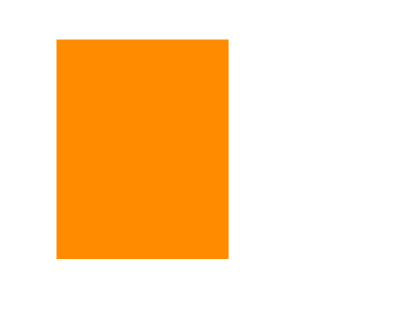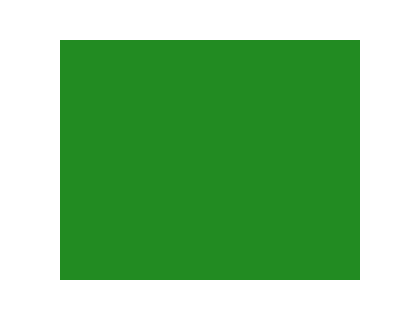# Math.random()

## Overview

The `Math.random()` function returns a random number between 0 and 1, including 0 but not including 1:

``Math.random();``

The `Math.random()` function is a method of the `Math` object, which has a set of functions that other programmers have defined for us to use. Here is a full list of available

## Generate a Random Number

In this example, we use the `Math.random()` function to generate a random number between 0 and 1 and assign it to the variable `x`. A new random number is generated each time the program is run.

``var x = Math.random();``

Quick Reference:

Message Log

## Scale a Random Number

The `Math.random()` function only generates random numbers between 0 and 1, but sometimes we need larger random numbers. We can create larger random numbers by multiplying values returned by the `Math.random()` function by a scale factor.

In this example, we generate random numbers between 0 and 50 by multiplying values returned by the `Math.random()` function by a scale factor of 50.

``var x = 50 * Math.random();``

Quick Reference:

Message Log

## Challenge 1Draw this square at a random position

Draw a square at a randomly generated position. Use the `Math.random()` function to generate a random value for the square's x-coordinate between 0 and 180 and a random value for the square's y-coordinate between 0 and 120.

Run your program a few times to make sure the square's position is randomly generated. If your program seems to be working, mark the challenge as complete by selecting "Yes, it looks good".

Quick Reference:

Message Log

## Generate a Random Number Within a Range

In this example, we generate random numbers between 40 and 120.

We multiply the values returned by the `Math.random()` function by a scale factor of 80, which is the difference between 120 and 40. This gives us random numbers between 0 and 80. Then, we add 40 to get random numbers between 40 and 120.

``var x = 40 + 80 * Math.random();``

Quick Reference:

Message Log

## Challenge 2Draw a rectangle with random dimensions at a random position

Draw a rectangle at a randomly generated position with randomly generated dimensions. Use the `Math.random()` function to generate a random value for the rectangle's x-coordinate between 20 and 160, a random value for the rectangle's y-coordinate between 20 and 60, and random values for the rectangle's width and height between 80 and 240.

Run your program a few times to make sure the rectangle's position and dimensions are randomly generated. Then, if your program seems to be working, mark the challenge as complete by selecting "Yes, it looks good".

Quick Reference:

Message Log

## Generate a Random Integer

There are a few ways to generate a random integer.

To generate a random integer between 0 and 9, we can generate a random number between 0 and 9, and then round the result using the `Math.round()` function. But, if we do that, we will generate only half as many 0's and 9's compared to the other numbers. This is because numbers between 0 and 0.5 will round to 0, but numbers between 0.5 and 1.5 (twice the range) will round to 1.

A better way to generate a random integer between 0 and 9 is to generate a random number between 0 and 10, and then round the result down using the `Math.floor()` function. This creates an even distribution of random integers, and we will not generate any 10's because `10 * Math.random()` is always less than 10 (remember, the `Math.random()` function returns values between 0 and 1, but not including 1).

``var x = Math.floor(10 * Math.random());``

To learn more about rounding numbers, visit the round() / floor() / ceil() lesson.

Quick Reference:

Message Log

## Challenge 3Fill this rectangle with a random color

Fill a rectangle with a randomly generated color. Use the `Math.random()` and `Math.floor()` functions to generate random RGB values (integers between 0 and 255) and assign them to three variables, `r`, `g`, and `b`. Those variables are used describe a color assigned to the `context.fillStyle` property.

Run your program a few times to make sure the rectangle's color is randomly generated. If your program seems to be working, mark the challenge as complete by selecting "Yes, it looks good".

If you need help defining fill colors, visit the fillStyle lesson.

Quick Reference:

Message Log

## Access a Random Element in an Array

In this example, we use the `Math.random()` function to access a random element in an array of New England state names.

``var states = ['Connecticut', 'Maine', 'Massachusetts', 'New Hampshire', 'Rhode Island', 'Vermont'];``

The elements in an array can be accessed by index. The first element in an array is at index 0 and the second element is at index 1. Since there are six elements in this array, we could generate a random index using `Math.floor(6 * Math.random())`, which generates a random integer between 0 and 5. The last index of an array with six elements is index 5 because the first index is 0.

However, it is generally not a good idea to assume that an array will always have six elements. Instead of hardcoding the number 6 in our random index generator, we can use the array's `length` property instead. Because the `states` array currently has six elements, `states.length == 6`. But if we add or remove elements later, the `length` property is automatically updated to reflect the new number. This makes using the array's `length` property to generate a random index much more robust than hardcoding a fixed number.

``````var i = Math.floor(states.length * Math.random()); // Generate a random index
var randomStateName = states[i]; // Use the random index to access a random element in the array``````

Try adding or removing names from the `states` array. The expression for generating a random index always selects an index in the appropriate range.

To learn more about variables and arrays, visit the Variables and Arrays lessons.

Quick Reference:

Message Log

## Challenge 4

Use a for loop to generate a random number of rectangles with randomly generated dimensions at randomly generated positions filled with a color randomly accessed from an array.

Assign a randomly generated integer between 4 and 10 to the variable `n`, which is the number of times the for loop will run.

Assign a randomly generated integer based on the `colors.length` property to the variable `i`, which is the index of the element in the `colors` array we will assign to the `context.fillStyle` property.

Inside the for loop, assign randomly generated numbers between 0 and 200 to the variables `x` and `y` for the position of the rectangle, and randomly generated numbers between 40 and 100 to the variables `w` and `h` for the dimensions of the rectangle.

Run your program a few times to make sure the number of rectangles, the color used to fill the rectangles, and each of the rectangle's dimensions and positions are randomly generated. Then, if your program seems to be working, mark the challenge as complete by selecting "Yes, it looks good".

Quick Reference:

Message Log